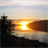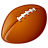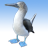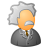# Moran's I computed in ArcGIS is different from that in R language (for same dataset)

3676
4
10-08-2012 02:15 PMNew Contributor
Hi,

I'm trying to get an understanding of global autocorrelation statistics by programatically implementing them.

I'm providing 10 rows of data given here  http://www.ats.ucla.edu/stat/r/faq/morans_i.htm, to ArcGIS (just considered Av8Top,Lat and Lon columns treating them as attribute value (which would be my input field for Moran's I computation), X and Y columns respectively) and then performed the Spatial Autocorrelation (spatial statistics toolbox).

Upon examining the results, the values generated in Arc (expected, observed, p-value and z-score) are not matching with those given on that page which are computed using R language. I have also written a simple java program computing Moran's I using the Global Moran's I explanation given in ArcGIS desktop help. They don't match them either.

It would be extremely helpful if some one can tell me if I'm missing any steps in arriving at the final values that are reported by the Spatial Autocorrelation tool and point me in the right direction.

Also it would be very helpful to have some sample datasets with precomputed autocorrelation statistic values so that I can tally my results with them.

Tags (3)
1 Solution

Accepted SolutionsNew Contributor III
I am the dev for the product.  Thanks to all for the replies....

Yes, if the weights are different, then the results are going to be different.  This is particularly evident with Inv Distance, as we apply a hybrid to avoid weights greater than 1... I.e. if dist < 1, then w = 1, else, w = 1/d^{exponent}

We also use the randomization assumption for the variance, but there is no "Monte Carlo".... That would be a "permutation" approach, which we do not use due to the extreme computational cost.

We also apply a "two-sided" alternative hypothesis... so, if you get the same weights into R in the form of a listw:

1. Make sure you honor the row standardization approach in both products
2. Try and use "Fixed Distance" to assure that our alternative IDW doesnt cause the issue
3. Make sure the alternative hypothesis is two.sided

I am attaching a zip file that contains CA counties and the SWM/GAL file necessary to compare.  Just run Moran inside ArcGIS using the caQueen.swm, and then run the R script to use spdep with caQueen.gal.  You will note that they are the same.  The image is in the zip file as well as below if you want to just take my word for it... but, the R script should show you how to call moran.test in a manner consistent with ArcGIS.

Thanks much,

MJ

[ATTACH=CONFIG]18654[/ATTACH]

[ATTACH=CONFIG]18653[/ATTACH]
4 RepliesOccasional Contributor III
Depending on how you specify your spatial weights matrix (Wij) the resulting Moran's-I can differ considerably. There is also the consideration of how you conducted the randomization in the Monte Carlo to ascertain significance. Without you describing how you conducted your analysis in both R (with code) and ArcGIS it is impossible to speculate on inconsistencies.New Contributor
Thank you for the response Sir.

I'm using inverse distance for the weighting in Arc as well as the java program. the R example given also uses inverse distance weighting. however i'm not sure if i'm explicitly using any Monte Carlo Randomization as ArcGIS Spatial Autocorrelation tool does not provide any option as such and in R language i haven't implemented the code, but I have compared the results given by Arc (for the same dataset) as specified @ http://www.ats.ucla.edu/stat/r/faq/morans_i.htm, may I know which randomization technique does Arc apply by default?New Contributor III
I am the dev for the product.  Thanks to all for the replies....

Yes, if the weights are different, then the results are going to be different.  This is particularly evident with Inv Distance, as we apply a hybrid to avoid weights greater than 1... I.e. if dist < 1, then w = 1, else, w = 1/d^{exponent}

We also use the randomization assumption for the variance, but there is no "Monte Carlo".... That would be a "permutation" approach, which we do not use due to the extreme computational cost.

We also apply a "two-sided" alternative hypothesis... so, if you get the same weights into R in the form of a listw:

1. Make sure you honor the row standardization approach in both products
2. Try and use "Fixed Distance" to assure that our alternative IDW doesnt cause the issue
3. Make sure the alternative hypothesis is two.sided

I am attaching a zip file that contains CA counties and the SWM/GAL file necessary to compare.  Just run Moran inside ArcGIS using the caQueen.swm, and then run the R script to use spdep with caQueen.gal.  You will note that they are the same.  The image is in the zip file as well as below if you want to just take my word for it... but, the R script should show you how to call moran.test in a manner consistent with ArcGIS.

Thanks much,

MJ

[ATTACH=CONFIG]18654[/ATTACH]

[ATTACH=CONFIG]18653[/ATTACH]New Contributor
Dear ESRI I have a question regarding the creation of a spatial weight matrix (SWM). I have a data set that contains 71 points depicting ozone values for two time periods (n = 142). When I create a SWM for each time period separately (i.e. independent of time) and run Moran�??s I I get the same results as my R Morans�?? I. That is ArcGIS and R produce the same results in Morans�?? I; hence, both methods must produce the same SWM. So this is a good check.

Now here is where things get interesting. When I create a SWM in ArcGIS using the concept of TIME_SPACE and run Morans�??I I get different results when compared to my R program using Moran�??s I. Since I have concluded that Moran�??s I is calculated the same in both my R program and ArcGIS and all the parameters are set the same in ARcGIS and in R the issue must lies in the generation of the SWM under the concept TIME_SPACE. So my questions is how does ArcGIS combine the matrices generated from the 71 points over two time periods. My SWM generated in R is 142 rows by 142 columns. If I take the ArcGIS SWM convert it to a tables I get 11534 records. If my guess is true (e.g. every table record is a element in the matrix) then ArcGIS does not create a symmetrical matrix. That is the root of 11534 is 107.4

In simple terms how are the intput matrix for the two time periods combine to create a single SWM under the TIME_SPACE concept.

Thanks
Mike